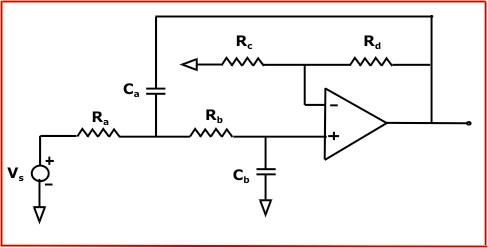Equal Component Butterworth Low Pass Filter Calculator Enter value of Cutoff Frequency, any one value - Resistor or Capacitor, any one value - Resistor Rc or Resistor Rd and click on calculate. Result will be displayed.Ra = Filter Resistor Rb = Filter Resistor (Ra = Rb) Ca = Filter Capacitor Cb = Filter Capacitor (Ca = Cb) Rc = Feedback Resistor Rd = Feedback Resistor

 Enter your values: Cutoff Frequency: Hz KHz MHz Results: (Enter any one value - Resistor or Capacitor and any one value - Resistor Rc or Resistor Rd) Resistor (Ra = Rb): ohm Kohm Mohm Capacitor (Ca = Cb): pF nF µF mF F Resistor Rc: ohm Kohm Mohm Resistor Rd: ohm Kohm Mohm

 Web www.calculatoredge.comLINKSDISCLAIMERCONTACT US